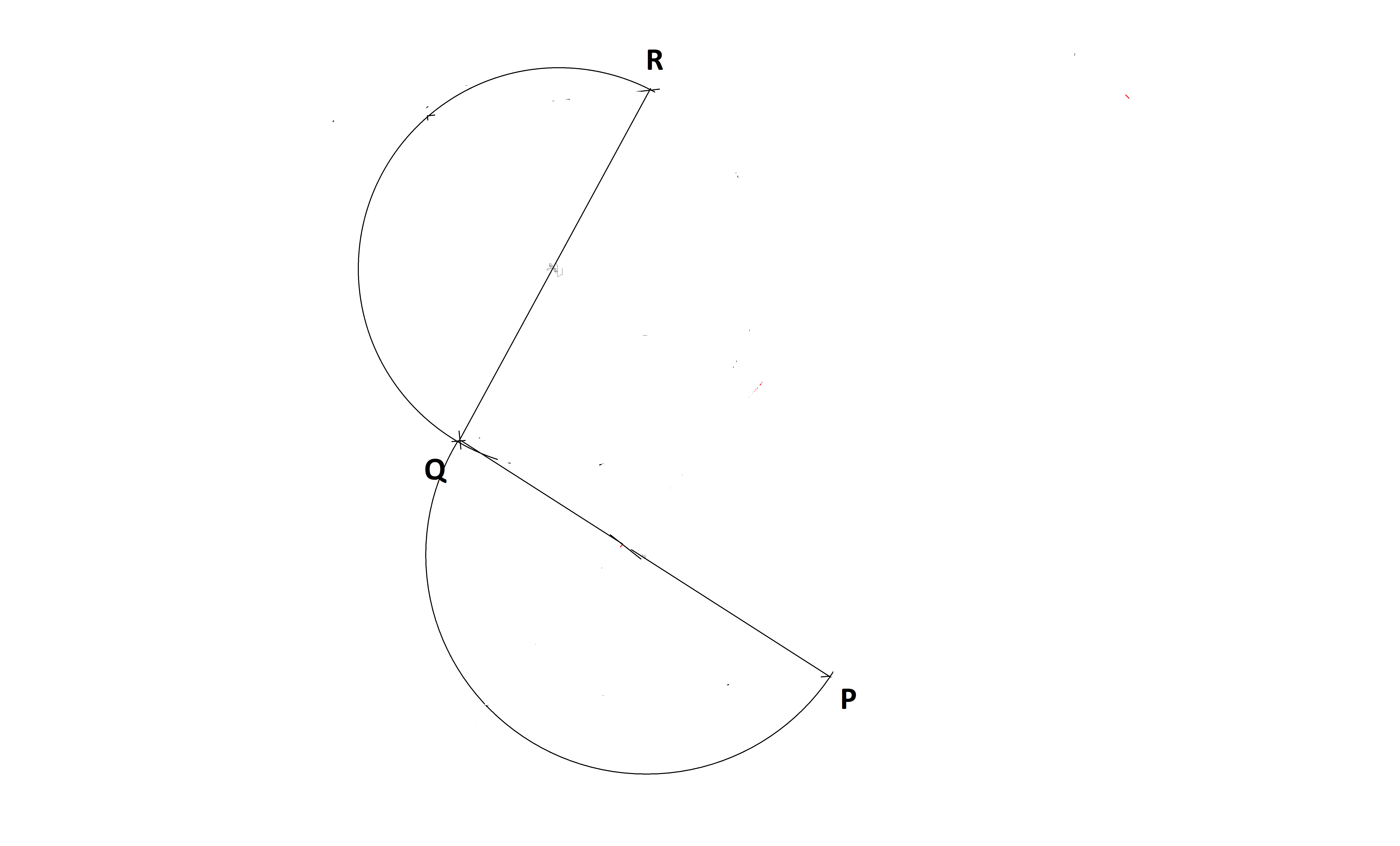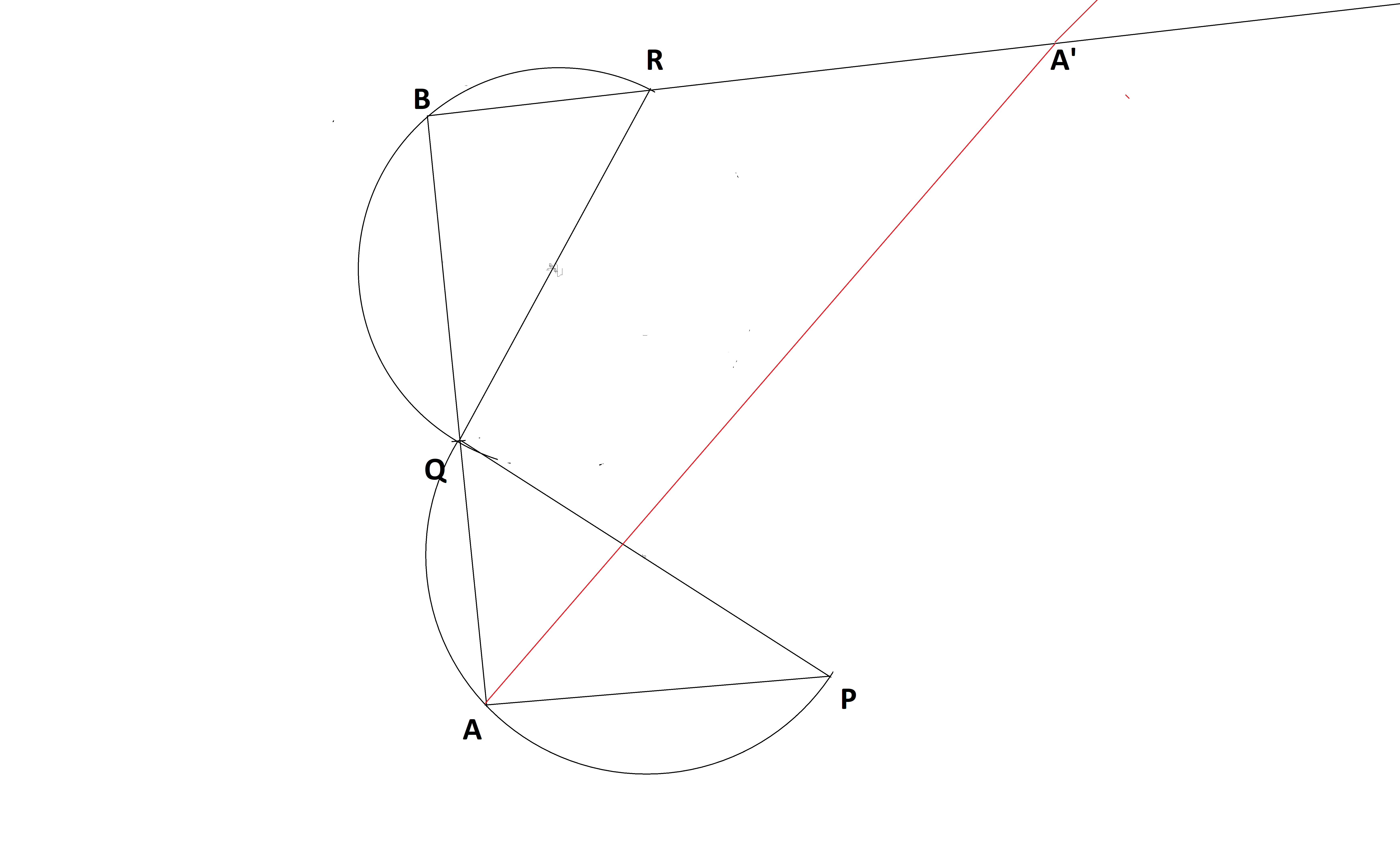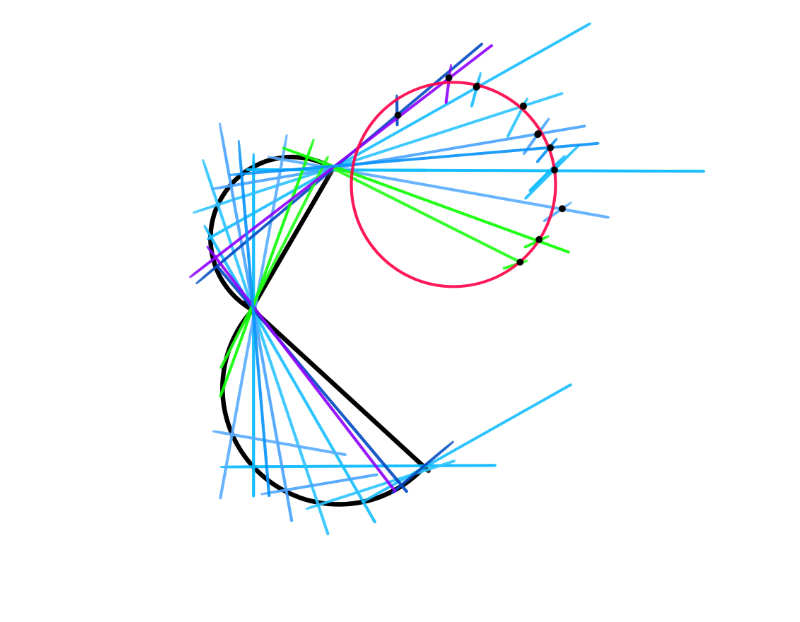# Got stuck at a point -3

While solving the four points on a square Bonus problem I was stuck at a last point (if someone solves this, then I can make a set of steps as an answer to the problem) please try to help.

Consider the following diagram ($0<\angle PQR < 180\degree$) :Let a process $P_1$ on a point $A$ that is on semicircle $QR$ be defined as follows :

• Join $\overline{AP}$ and $\overline{AQ}$

• Extend $\overline{AQ}$ till it intersects the semicircle $RQ$ at a point B

• Join $\overline{RB}$ and extend

• Draw the angular bisector of $\angle PAQ$ and let it intersect extended $\overline{RB}$ at point $A'$

• Let $A'$ be called as the output of the process

An illustration of process $P_1$ on a point $A$ on the semicircle $QR$ ($\red{red}$ line is the angular bisector) :Now imagine that you does this process on every point of the semicircle $QP$ and marks each and every output, what will be the shape made by those outputs? (A circle, A parabola, A square...)

Note:

• Any point on the diameter is not counted as a point on the semicircle

• If anyone gives the answer with a proof, I will immediately write a solution to the four point in square problem as I am just one solution far to the problem (that is this problem)Note by Zakir Husain
1 year ago

This discussion board is a place to discuss our Daily Challenges and the math and science related to those challenges. Explanations are more than just a solution — they should explain the steps and thinking strategies that you used to obtain the solution. Comments should further the discussion of math and science.

When posting on Brilliant:

• Use the emojis to react to an explanation, whether you're congratulating a job well done , or just really confused .
• Ask specific questions about the challenge or the steps in somebody's explanation. Well-posed questions can add a lot to the discussion, but posting "I don't understand!" doesn't help anyone.
• Try to contribute something new to the discussion, whether it is an extension, generalization or other idea related to the challenge.

MarkdownAppears as
*italics* or _italics_ italics
**bold** or __bold__ bold
- bulleted- list
• bulleted
• list
1. numbered2. list
1. numbered
2. list
Note: you must add a full line of space before and after lists for them to show up correctly
paragraph 1paragraph 2

paragraph 1

paragraph 2

[example link](https://brilliant.org)example link
> This is a quote
This is a quote
    # I indented these lines
# 4 spaces, and now they show
# up as a code block.

print "hello world"
# I indented these lines
# 4 spaces, and now they show
# up as a code block.

print "hello world"
MathAppears as
Remember to wrap math in $$ ... $$ or $ ... $ to ensure proper formatting.
2 \times 3 $2 \times 3$
2^{34} $2^{34}$
a_{i-1} $a_{i-1}$
\frac{2}{3} $\frac{2}{3}$
\sqrt{2} $\sqrt{2}$
\sum_{i=1}^3 $\sum_{i=1}^3$
\sin \theta $\sin \theta$
\boxed{123} $\boxed{123}$

Sort by:

Have a look at this, it might help :)- 1 year ago

Wow,how do you make it.. @Jeff Giff

Sketchbook, iPad. Designed for art, but works well in these situations :)

- 1 year ago

I had also tried to make this and i also got an approximated circle, but I need a proof for that.

- 1 year ago

Yes,math requires proof.I am also trying to prove.

Are QR and QP perpendicular to each other ?? Please reply @Zakir Husain

No they are at an arbitrary angle

- 1 year ago

Oooooooo ,

Hint: Complete the semicircles. Call the midpoint of arc $PQ$(the one that the angle bisector will pass through) $M$, and the two completed circle intersect at another point $Q'$. You can try to prove that $R,A',Q',M$ are concyclic. (Since $R,Q',M$ are fixed points, this leads to the locus of $A'$ being a circle. A simple angle chasing will prove this. Actually, this is just a special case of Miquel's theorem, or Pivot theorem, so the proof is the same.)

- 1 year ago

Hope this hint helps, and I'm looking forward to see your solution to the four point in square problem !

- 1 year ago

Yes,it helps.....

I have only 1 doubt, what is the angle between seg QP and seg QR, I know it has to be greater than 0 degrees and lesser than 180 degrees, otherwise getting point B is not possible on the other semicircle, unless the point A coincides with the endpoints of the diameter or is on the diameter, which you have restricted from doing so. Also not greater than 180, because the semicircles would overlap then which I assume is not allowed.

$0<\angle PQR<\pi$

- 1 year ago

Yes that is what I meant, thank you! I will work on the proof now and see if I can do it.👍

- 1 year ago

- 1 year ago